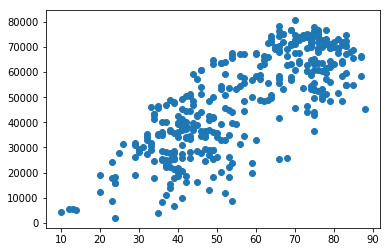## Notes for March 21¶

What factors predict the number of Citi Bike rides in NYC? In this lesson we'll use a linear regression model to guess. Note that although it's hard not to talk about this case in causal terms ("rain causes fewer rides"), there's nothing about the mathematical model that implies causation: we could just as easily predict rain given rides.

In :
import numpy
import pandas
from sklearn.linear_model import LinearRegression
from matplotlib import pyplot

In :
citibike = pandas.read_csv("citibike_weather.csv")

In :
citibike.describe()

Out:
trips max_temp min_temp avg_temp departure_from_norm heating cooling precip snow
count 365.000000 365.000000 365.000000 365.000000 365.000000 365.000000 365.000000 365.000000 365.000000
mean 48077.641096 62.643836 49.263014 56.205479 1.194521 12.720548 3.926027 0.180521 0.108904
std 19453.477478 18.410736 17.395429 17.741860 7.952329 13.371026 5.986019 0.399386 0.846831
min 1922.000000 13.000000 5.000000 10.000000 -23.000000 0.000000 0.000000 0.000000 0.000000
25% 33149.000000 47.000000 35.000000 41.000000 -4.000000 0.000000 0.000000 0.000000 0.000000
50% 49201.000000 62.000000 47.000000 54.000000 1.000000 11.000000 0.000000 0.010000 0.000000
75% 65887.000000 79.000000 66.000000 73.000000 6.000000 24.000000 8.000000 0.110000 0.000000
max 80651.000000 96.000000 81.000000 88.000000 31.000000 55.000000 23.000000 2.900000 9.800000

It's pretty clear that there's a relationship between average temperature and rides.

In :
pyplot.scatter(citibike.avg_temp, citibike.trips)
pyplot.show()Can we quantify that? Let's look at covariance. It's big, but covariance scales with the units we're measuring, and rides are in the tens of thousands per day.

In :
temp_trips = numpy.cov(citibike.avg_temp, citibike.trips)
temp_trips

Out:
array([[3.14773596e+02, 2.69179071e+05],
[2.69179071e+05, 3.78437786e+08]])

Dividing by the product of the standard deviations scales the covariance down to a more interpretable number, the correlation. That looks pretty high.

In :
temp_trips[0,1] / numpy.sqrt( temp_trips[0,0] * temp_trips[1,1] )

Out:
0.7799107307679495

Alternatively, dividing the covariance by the variance of one variable gives us the estimated slope of a linear model. This result means that if the temperature increases by one degree fahrenheit, we expect about 850 more rides.

In :
temp_trips[0,1] / temp_trips[0,0]

Out:
855.1513671898349

### Confusing but important interlude¶

Pandas DataFrame objects consist of a set of named columns. There are several ways of asking for one or more columns. They result in numpy arrays of different shapes.

In :
citibike.trips.shape

Out:
(365,)
In :
citibike["trips"].shape

Out:
(365,)
In :
citibike[ ["trips", "avg_temp", "precip"] ].shape

Out:
(365, 3)
In :
citibike[ ["trips"] ].shape

Out:
(365, 1)

The first treated "trips" as an attribute of an object. The second was a more array-like syntax. Both return a one-axis array (like a list). We can also use the same syntax to ask the data frame for an array containing multiple field names, and get out an array with two axes (like a table). Using that "double array" syntax with only one field name gives us the same values as asking just for that field, but in a two-axis table that just happens to only have one column.

Why do we care? Because if we pass a one-axis array to the LinearRegression object, it gives us the world's scariest error message.

Here's what it looks like when it works.

In :
temp_model = LinearRegression().fit(citibike[["avg_temp"]],
citibike[["trips"]])


The coefficients of the fitted model give us the optimal slope for the linear model. This number should look familiar!

In :
temp_model.coef_

Out:
array([[855.15136719]])

The reason I'm bringing in SciKit packages rather than just dividing the covariance by the variance is that I can now ask for coefficients for more than one variable simultaneously.

If we add precipitation, we find that one additional inch of precipitation (a major rain event, or about 10" of snow) is associated with 15,000 fewer trips.

Look also at what happened to the coefficient for temperature. It's about the same, slightly higher. Most days don't have rain, but those that do are way below. If the model doesn't know which days have rain, it's going to have a flatter slope for temperature, because otherwise warm days will have low numbers of trips. Since the precipitation coefficient is now accounting for the fact that those days will be lower, we're free to increase the temperature coefficient.

In :
t_p_model = LinearRegression().fit(citibike[["avg_temp", "precip"]],
citibike[["trips"]])
t_p_model.coef_

Out:
array([[   870.18184118, -15967.91988972]])

Sometimes useful information doesn't come in number form. In this case it's often helpful to define "dummy" or "indicator" variables. Here we'll create a variable that is 1 if the day is a weekend and 0 if it is a weekday.

Indicator variables wouldn't make much sense as a single linear predictor: we could just divide the data into two sections and calculate the mean for each. But in the context of multiple inputs for linear regression, they are very useful.

In :
def is_weekend(s):
if s == "Saturday" or s == "Sunday":
return 1
else:
return 0

In :
is_weekend("Sunday")

Out:
1
In :
citibike["weekend"] = citibike.weekday.map(is_weekend)

Out:
0    0
1    0
2    0
3    0
4    0
5    1
6    1
7    0
8    0
9    0
Name: weekend, dtype: int64

Here's a model with four inputs and an intercept. Each additional variable seems to add something. As we increase the number of inputs, reasoning about the effect of each variable on the coefficients of the other variables becomes difficult. The temperature coefficient is now lower, but I have a harder time telling a story about why.

In :
predictors = ["avg_temp", "precip", "weekend", "snow"]
model = LinearRegression().fit(citibike[predictors],
citibike[["trips"]])
model.coef_

Out:
array([[   855.01538303, -15321.55233555,  -9370.35853807,
-1047.28690233]])

Does this model make sense in the extremes? This says that a 0-degree weekday with no rain or snow should have 5571 rides. Add half an inch of precipitation and we would get -2000 rides. Does this matter? It depends on how we are using the model.

In :
model.intercept_

Out:
array([5570.91080233])